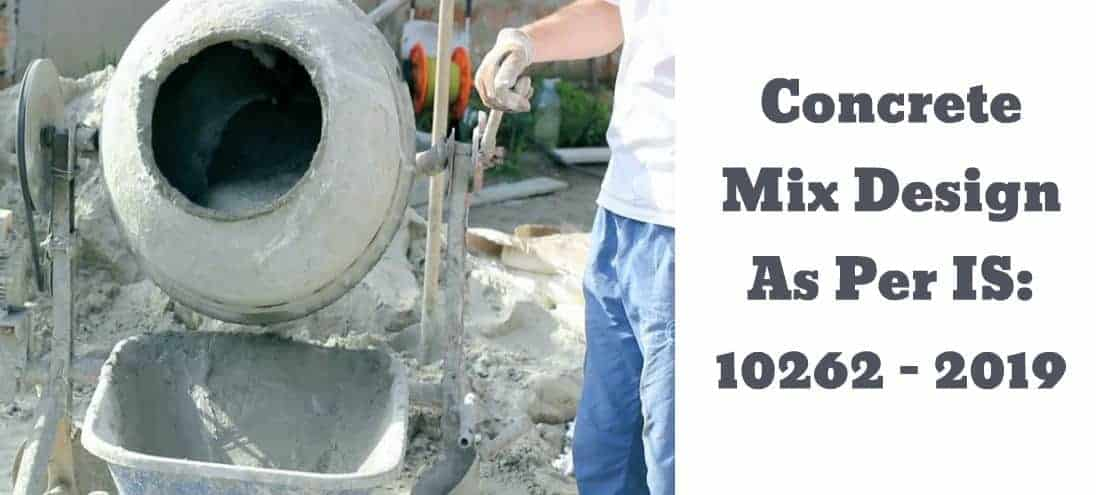# Mix Design of Concrete For M20, M25, and M30 With Procedure9 min read

Post Contents

## Mix Design of Concrete

Mix Design of Concrete is the process of determining the right proportions of cement, sand, and aggregates for concrete to achieve the target strength of concrete.

The Advantage of concrete mix design is that it gives the right proportions of materials, thus making the concrete use economical in achieving the required strength of structural members.

As, the quantity of concrete required for huge, constructions are huge, the economy in the number of materials such as cement makes the project construction economical.

## Concrete Mix Design Example

Following is a Mix Design of Concrete example

### Data Required Mix Design

#### i.Design Data

(a) Characteristic compressive strength of concrete required at end of 28 days  = M 25

(b) Nominal maximum size of aggregate used = 20 mm

(c) Shape of Coarse Aggregate = Angular

(d) Required workability at site = 50-75 mm (slump Value)

(e) Quality control is done by as per IS: 456

(f) Type of exposure Condition of concrete (as defined in IS: 456) = Mild

(g) Type of cement used = PSC conforming IS: 456 – 2000

(h) Method of placing Concrete on Site = pumpable concrete

#### (ii) Material testing data (determined in the laboratory)

(a) Specific gravity of cement =  3.15

(b) Specific gravity of FA = 2.64

(c) Specific gravity of CA = 2.84

(d) Aggregates are assumed to be having surface dry conditions.

(e) Fine aggregates are confirmed to Zone II of IS – 383

## Mix Design of ConcreteProcedure

The following steps to be followed for concrete mix design calculation,

### Step-1 Determining the Target Strength of Concrete

Himsworth constant for 5% risk factor is 1.65 & standard deviation is taken from IS: 456 2000 for M – 25 Grade of concrete is  4.0.

ftarget = fck + 1.65 x S

= 25 + 1.65 x 4.0 = 31.6 N/mm2

Where,

S = Standard Deviation (N/mm2) = 4 (Refer: IS 10262- 2009 table -1)

### Step-2 Determining Water-Cement ratio

Refer:  IS 456, (page no 20) Table 5

Maximum water-cement ratio taken for Mild exposure condition = 0.55

Assume watercement ratio as 0.50 for experiment base

0.5 < 0.55, hence it is OK.

Read More: Concrete Mix Design Calculations and Its Ratio for M 25 Grade

### Step-3 Selection of Water Content for Mix

Refer: IS 10262- 2009 Table 2

Maximum water content is taken = 186 Kg (maximum size of aggregate = 20 mm)Correction in water content is

Estimated water content for mix = 186+ (3/100) x 186 = 191.6 kg /m3

### Step-4 Selection of Cement Content for Mix

• Water-cement ratio = 0.50
• Corrected water content for mix = 191.6 kg /m3
• Cement content =
• Refer IS 456 – 2000, Table 5
• Minimum cement Content required for mild exposure condition = 300 kg/m3
• 383.2 kg/m3 > 300 kg/m3, hence, OK.
• This value is required to be checked for durability requirement from IS: 456 – 2000
• In this example for mild exposure and for the reinforced concrete the minimum cement content is 300 kg/m3 which are less than 383.2 kg/m3. Hence cement content adopted = 383.2 kg/m3.
• IS: 456: 2000, clause 8.2.4.2
• Maximum cement content for mix = 450 kg/m3.

### Step-5 Estimation of Coarse Aggregate Proportion for Mix

Refer IS 10262- 2009, Table 3

• For Nominal max. size of aggregate for mix = 20 mm,
• Zone of fine aggregate = Zone II
• And For w/c = 0.5
• The volume of coarse aggregate per unit volume Concrete = 0.62

#### Table for Correction in Estimation of Coarse Aggregate Proportion

(Note 1: For every ±0.05 increase or decrease change in w/c, the coarse aggregate proportion is to be changed by 0.01. If the w/c is less than 0.5, the volume of coarse aggregate is required to be increased to reduce the fine aggregate content in the mix. If the water-cement ratio is more than 0.5, the volume of coarse aggregate is to be reduced to increase the fine aggregate content. If the coarse aggregate is not of an angular shape, the volume of coarse aggregate is required to be increased suitably, based on experience-based.)

(Note 2: For pumpable concrete or congested reinforcement in structure the coarse aggregate proportion may be reduced up to 10%)

Hence,Volume of coarse aggregate per unit volume of total Volume of concrete = 0.62 x 90% = 0.558

Volume of fine aggregate in mix = 1 – 0.558 = 0.442

### Step-6 Estimation of the Mix Materials

Volume of concrete is taken = 1 m3

Volume of cement = (Weight of cement / Specific gravity of cement) x (1/100) = (383.2/3.15) x (1/1000) = 0.122 m3

Volume of water = (Weight of water / Specific gravity of water) x (1/1000)

= (191.6/1) x (1/1000) = 0.1916 m3

Volume of aggregates = a – (b + c ) = 1 – (0.122 + 0.1916) = 0.6864 m3

Weight of coarse aggregates = 0.6864 x 0.558 x 2.84 x 1000 = 1087.75 kg/m3

Weight of fine aggregates = 0.6864 x 0.442 x 2.64 x 1000 = 800.94 kg/m3

### Concrete Mix Proportions for Concrete Trial Mix -1

• Cement = 383.2 kg/m3
• Water = 191.6 kg/m3
• Fine aggregates = 800.94 kg/m3
• Coarse aggregate = 1087.75 kg/m3
• W/c = 0.5
• For trial -1 of concrete in lab & to check its properties.
• It will satisfy durability & economy.
• For making trial -1, mass of ingredients required will be calculated for 4 no’s cube assuming 25% wastage.
• Volume of concrete required for Making 4 cubes = 4 x (0.15x1.25) = 0.016878 m3
• Wt. of Cement = (383.2 x 0.016878) kg/m3 = 6.47 kg
• Wt. of Water = (191.6 x 0.016878) kg/m3 =3.23 kg
• Wt. of Coarse aggregate = (1087.75 x 0.016878) kg/m3 =18.36 kg
• Wt. of Fine aggregates = (800.94 x 0.016878) kg/m3 = 13.52 kg

### Step-7 Correction for Absorption / Moisture of Aggregate

As we assumed the aggregate is a saturated surface dry condition, therefore no correction is required.

### Step-8 Concrete Trail Mixes

##### Concrete Trial Mix 1
• The mix proportion determined in Step 6 Make trial mix -1 & with this proportion, concrete is manufactured and tested for fresh concrete properties requirements like workability, bleeding, and finishing qualities.
• In this Trial Mix,
• Slump value = 25 mm
• Compaction Factor = 0.844
• From the Mix Slum test, the concrete is workable and had a true slump of about 25 mm and it is free from segregation and bleeding.
• required slump = 50-75 mm
• So modifications and changes are needed in trial mix 1 to get the desired workability.

### Concrete Trial Mix 2

• To increase the workability of concrete mix from 25 mm to 50-75 mm an increase in water content by +3% is to be made.
• The corrected water content for mix = 191.6 x 1.03 = 197.4 kg.
• As mentioned earlier to adjust fresh concrete properties the water cement ratio should not be changed. Hence
• Cement Content = (197.4/0.5) = 394.8 kg/m3
• Which also need to satisfy durability requirement.
• Volume of aggregate in Concrete = 1 – [{394.8/(3.15×1000)} + {197.4/(1 x 1000)}] = 0.6773 m3
• Weight of coarse aggregate = 0.6773 x 0.558 x 2.84 x 1000 = 1073.33 kg/m3
• Weight of fine aggregate = 0.6773 x 0.442 x 2.64 x 1000 = 790.3 kg/m3

### Concrete Mix Proportions for Trial Mix 2

• Weight of Cement = 384.8 kg/m3
• Weight of  Water = 197.4 kg/m3
• Weight of  Fine aggregate =790.3 kg/m3
• Weight of Coarse aggregate = 1073.33 kg/m3
• For making trial -2, the Weight of material required will be calculated for 4 no’s cube assuming 25% wastage.
• Volume of concrete required for 4 cubecasting = 4 x (0.153 x1.25) = 0.016878 m3
• Weight of Cement = (384.8 x 0.016878) kg/m3 = 6.66 kg
• Weight of Water = (197.4 x 0.016878) kg/m3 =3.33 kg
• Weight of Coarse aggregate = (1073.33 x 0.016878) kg/m3 =18.11 kg
• Weight of Fine aggregates = (790.3 x 0.016878) kg/m3 = 13.34 kg
• In this trial Mix,
• Slump value for mix = 60 mm
• Compaction Factor for mix = 0.852
• So, from the results of the slump of Mix, the concrete is workable and had a true slump of about 60 mm.
• Required slump = 50-75 mm
• So, it satisfied the desired workability by satisfying the requirement of 50-75 mm slump value.
• Now, we have to go for trial mix-3

### Concrete Trial Mix 3

• In this trial mix water/cement ratio is to be decreased by 10% keeping water content constant.
• Water cement ratio = 0.45
• With the reduction of 0.05 in w/c, we have to increase of coarse aggregate fraction by 0.01.
• Coarse aggregate fraction in mix = 0.558 +0.01 =0.568
• W/c = 0.45 and water content for mix = 197.4 kg/m3
• Cement content for mix = (197.4/0.45) = 438.7 kg/m3
• Volume aggregate in total volume of concrete = 1 – [{438.7/(3.15 x 1000)} + (197.4/1000)] = 0.664 m3
• Weight of coarse aggregate = 0.664 x 0.568 x 2.84 x 1000 = 1071.11 kg/m3
• Weight of fine aggregate = 0.664 x 0.432 x 2.64 x 1000 = 757.28 kg/m3

## M 25 Mix Design of Concrete

For the Compressive Strength of concrete vs. C/W graph for target strength 31.6 MPa, we found

• Water cement ratio = 0.44
• Weight of water content = 197.4 kg/m3
• Weight of Cement content = (197.4/0.44) = 448.6 kg/m3
• Volume of aggregate in total volume of concrete = 1 – [{448.6/(3.15 x 1000)} + (197.4/1000)] = 0.660 m3
• A reduction of 0.05 in w/c, we have to increase of coarse aggregate fraction by 0.01.
• Coarse aggregate volume = 0.558 +0.01 =0.568 m3
• Volume of fine aggregate in mix = 1 – 0.568 = 0.432 m3
• Weight of coarse aggregate = 0.660 x 0.568 x 2.84 x 1000 = 1064.65 kg/m3
• Weight of fine aggregate = 0.660 x 0.432 x 2.64 x 1000 = 752.71 kg/m3

## FAQs

### What are the requirements of concrete mix design?

Requirements of concrete mix design are listed below:
1. Characteristic compressive strength of concrete
2. Nominal maximum size of aggregate used
3. Shape of Coarse Aggregate
4. Required workability at site
5. Quality control is done by as per IS: 456
6. Type of exposure Condition of concrete
7. Type of cement used

### What is Concrete Mix Design?

Mix Design of Concrete can be defined as the method of calculating a suitable quantity of materials of concrete and finding out the required proportions with the object of producing concrete of certain minimum strength and durability as economically as possible.

### What is IS code for concrete mix design?

The Bureau of Indian Standards recommended a set procedure for the design of concrete mix mainly based on the work done in national laboratories. The Concrete Mix Design Calculation procedure is covered in IS 10262: 2019.

### How is concrete mix design calculated?

The following Steps Should be followed to calculate Concrete Mix Design:
Step 1: Determining the Target Strength of Concrete
Step 2 Determining Water-Cement ratio
Step 3: Selection of Water Content and Cement Content for Mix
Step 4: Estimation of Coarse Aggregate Proportion for Mix
Step 5: Correction for Absorption and Concrete Trail Mixes

### What is M25 concrete mix design?

M-25 MIX DESIGNS (as per IS-10262-2009):
M25 is a grade designation, having a Maximum nominal crushed angular aggregate size around 20 mm, whose workability lies within 50-75 mm and is categorized under the type ‘good degree of supervision.

### What is the strongest concrete mix ratio?

Concrete Mix Ratio of 1:3:5,
Concrete Mix Ratio 1:3:5 is considered to be a strong concrete mix design. Where 1:3:5 complies Cement: Sand: Coarse Gravel.

You May Also Like

### 1 thought on “Mix Design of Concrete For M20, M25, and M30 With Procedure”

1.Concrete Mix Design

### Popular Post on Website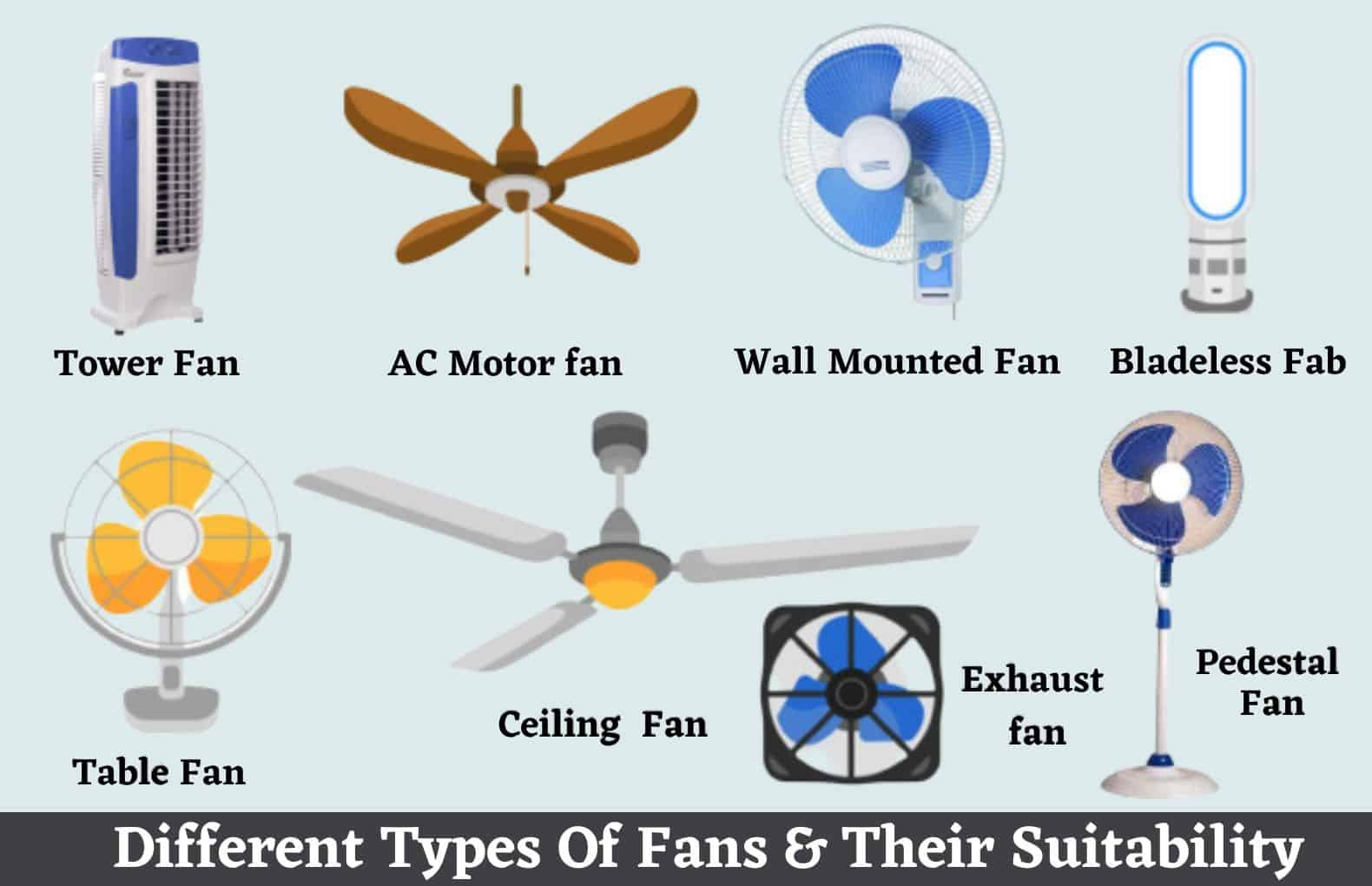#### 15 Types of Fans | Different Types of Fans For Home | Ceiling Fan Type | Best Fan Types & Their Uses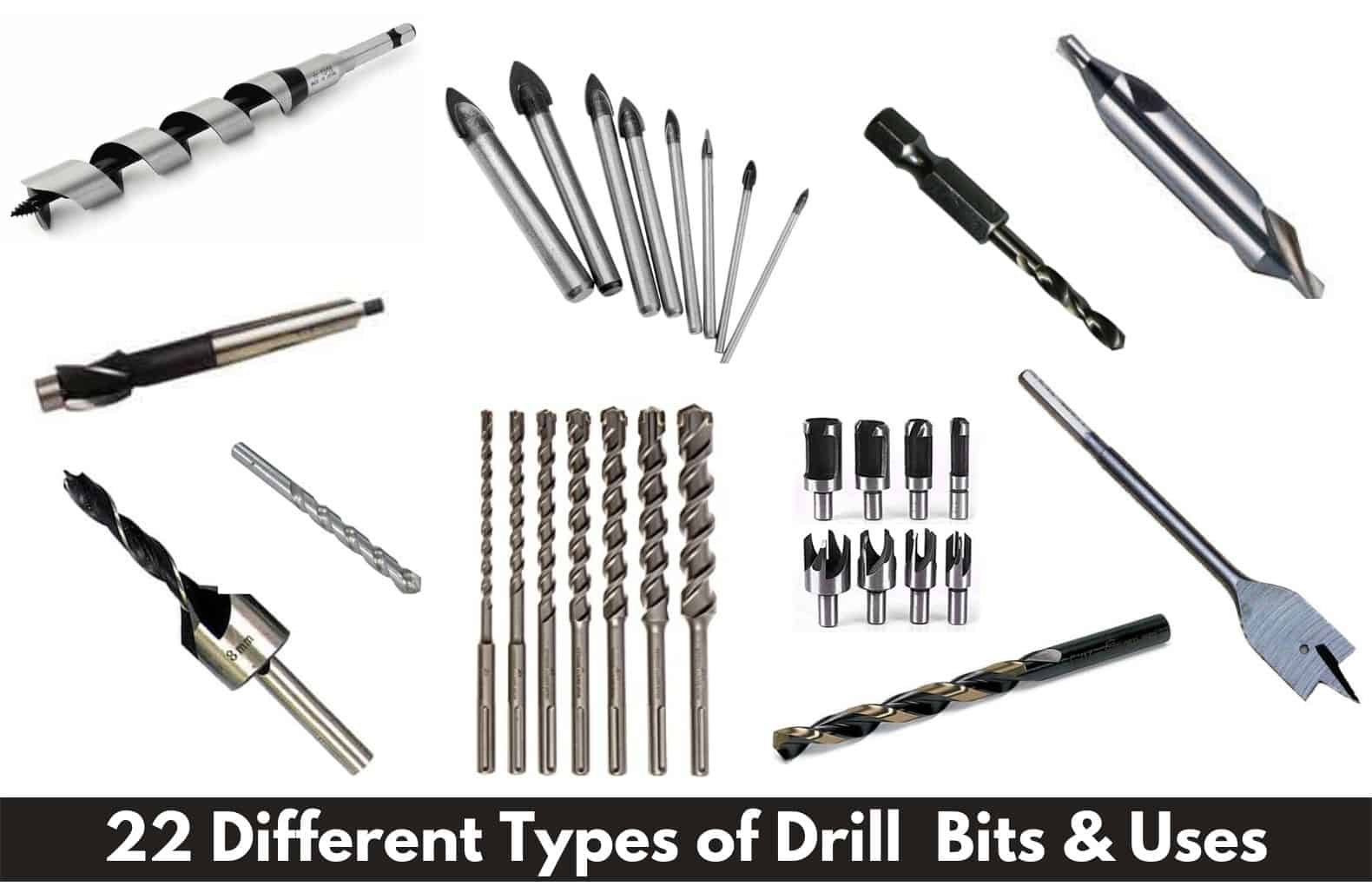#### 22 Types of Drill Bits | Drill Bit Types And Uses | Different Drill Bits Name | Best Drill Bits For Cutting Work#### 40 Types Of Tables | Different Types of Tables | Best Types Of Table For Living Room | Different Kinds Of Tables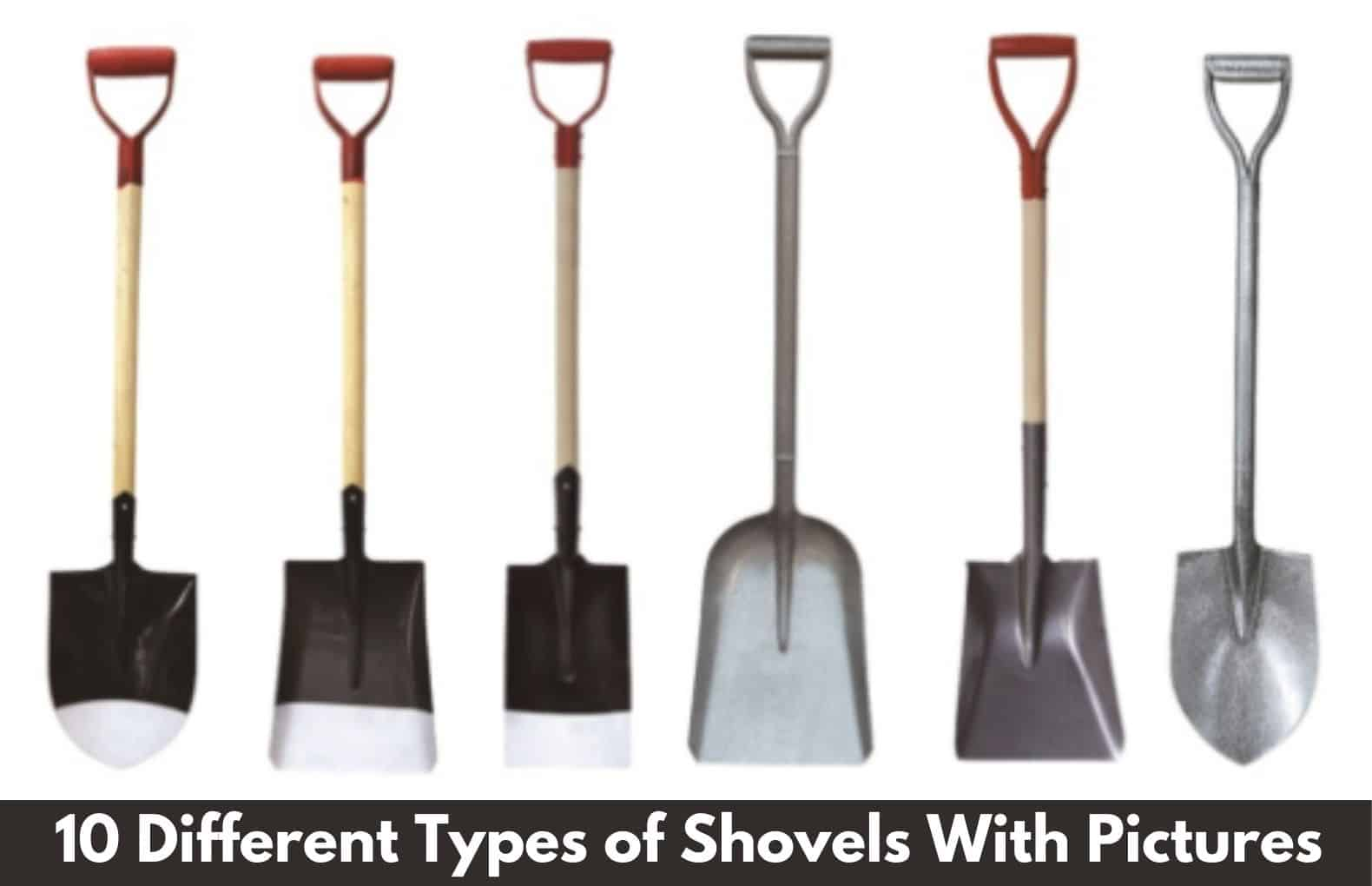#### 10 Types of Shovels | Different Types of Shovels With Pictures | Best Shovels For Digging Work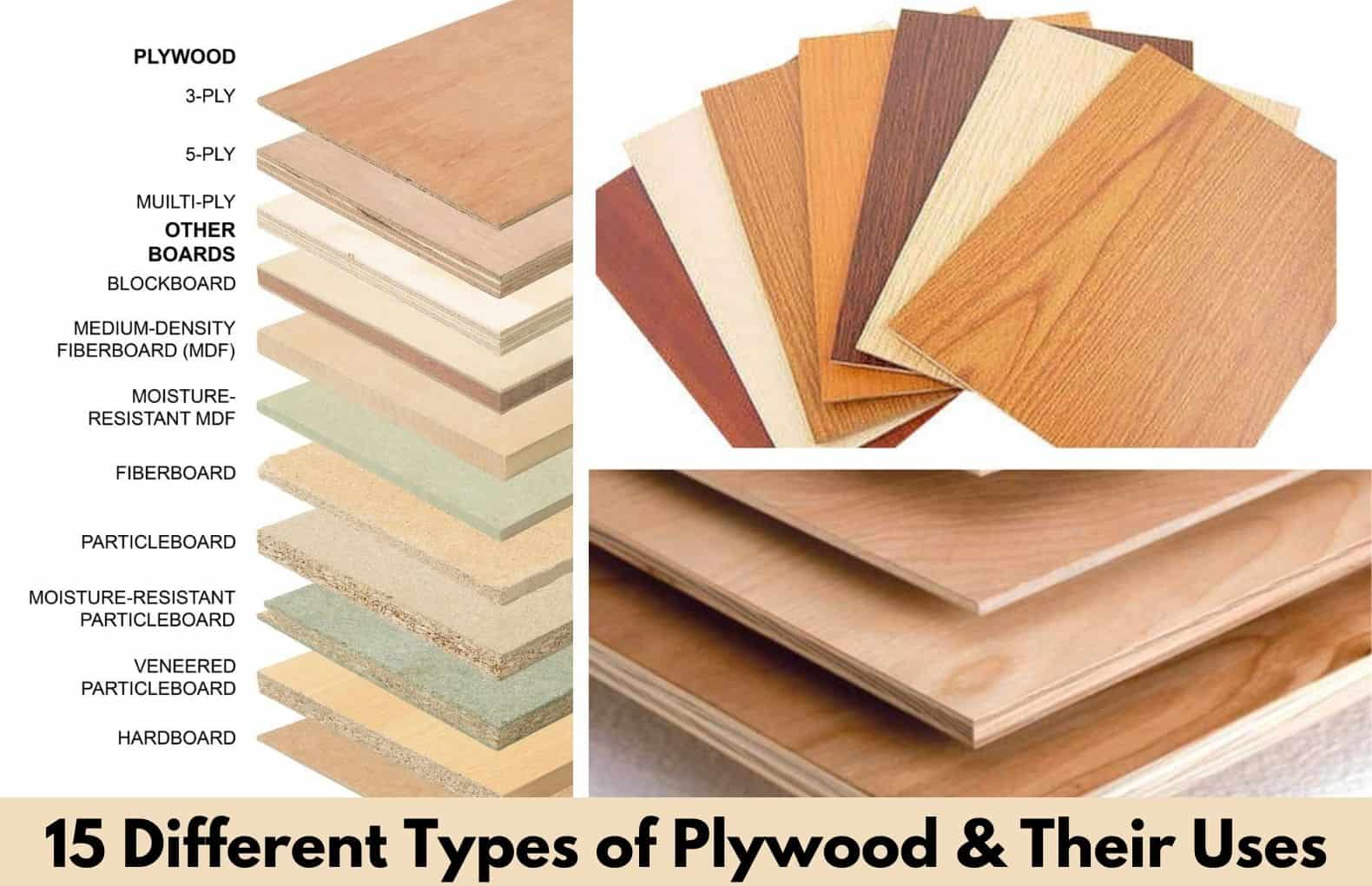#### 15 Different Types of Plywood | What Is Plywood and Their Types | 15 Best Types of Plywood for Furniture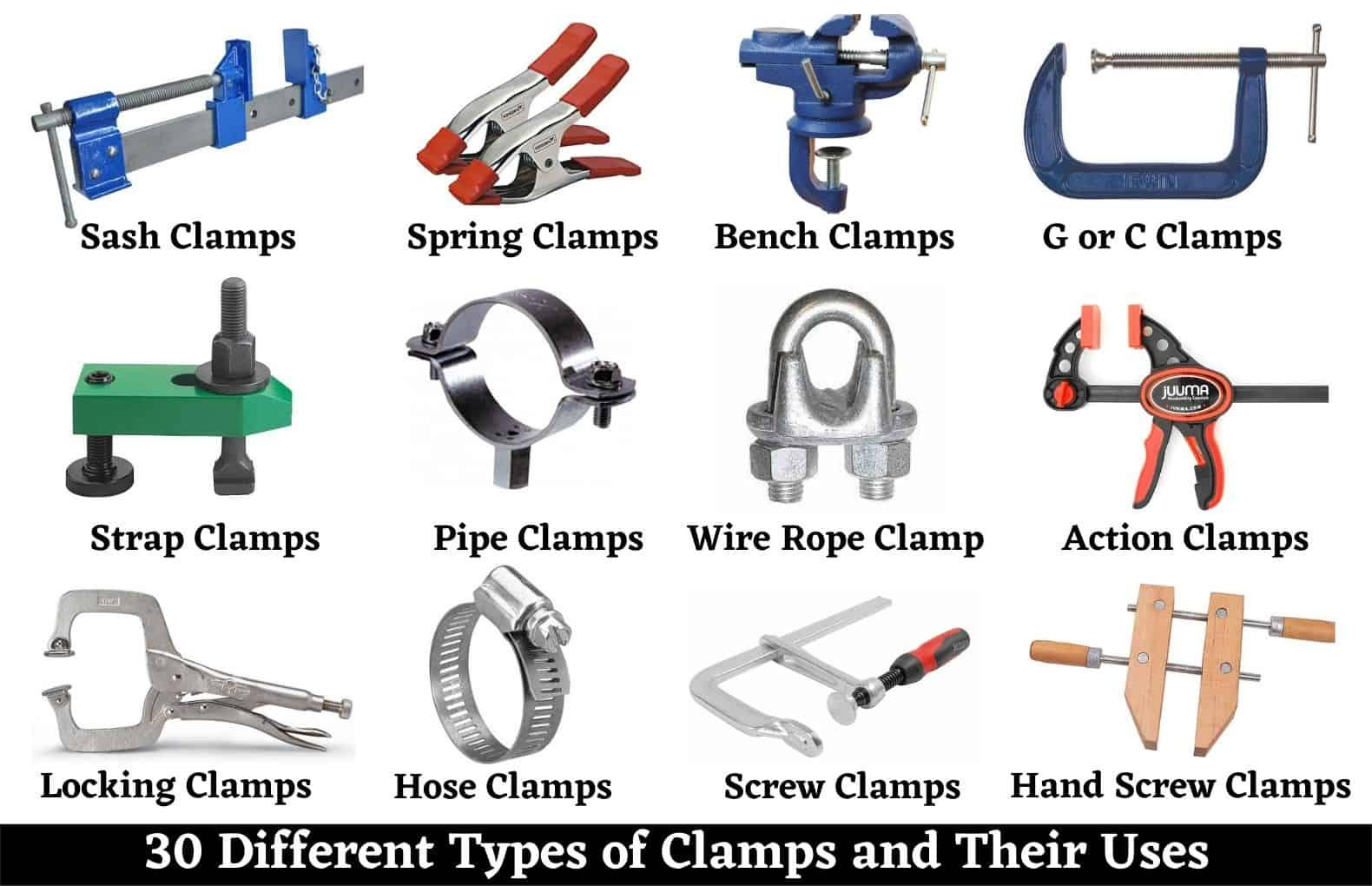#### 30 Types of Clamps and Their Uses | 30 Different Types Clamps and Uses | Best Clamps For Home Uses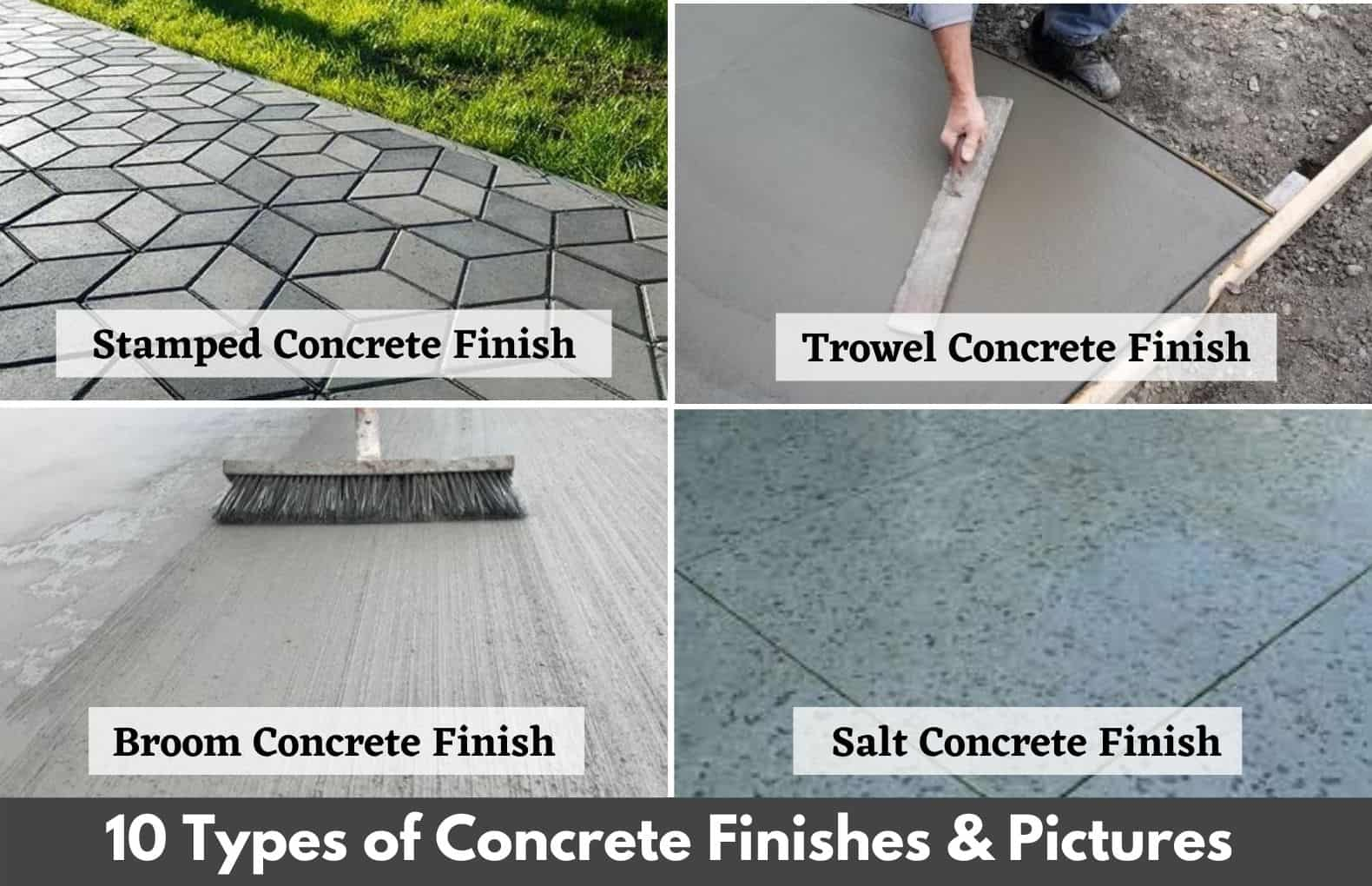#### 10 Concrete Finishes Types | 10 Best Types of Concrete Finishes | Different Concrete Finishes | Concrete Surface Finishes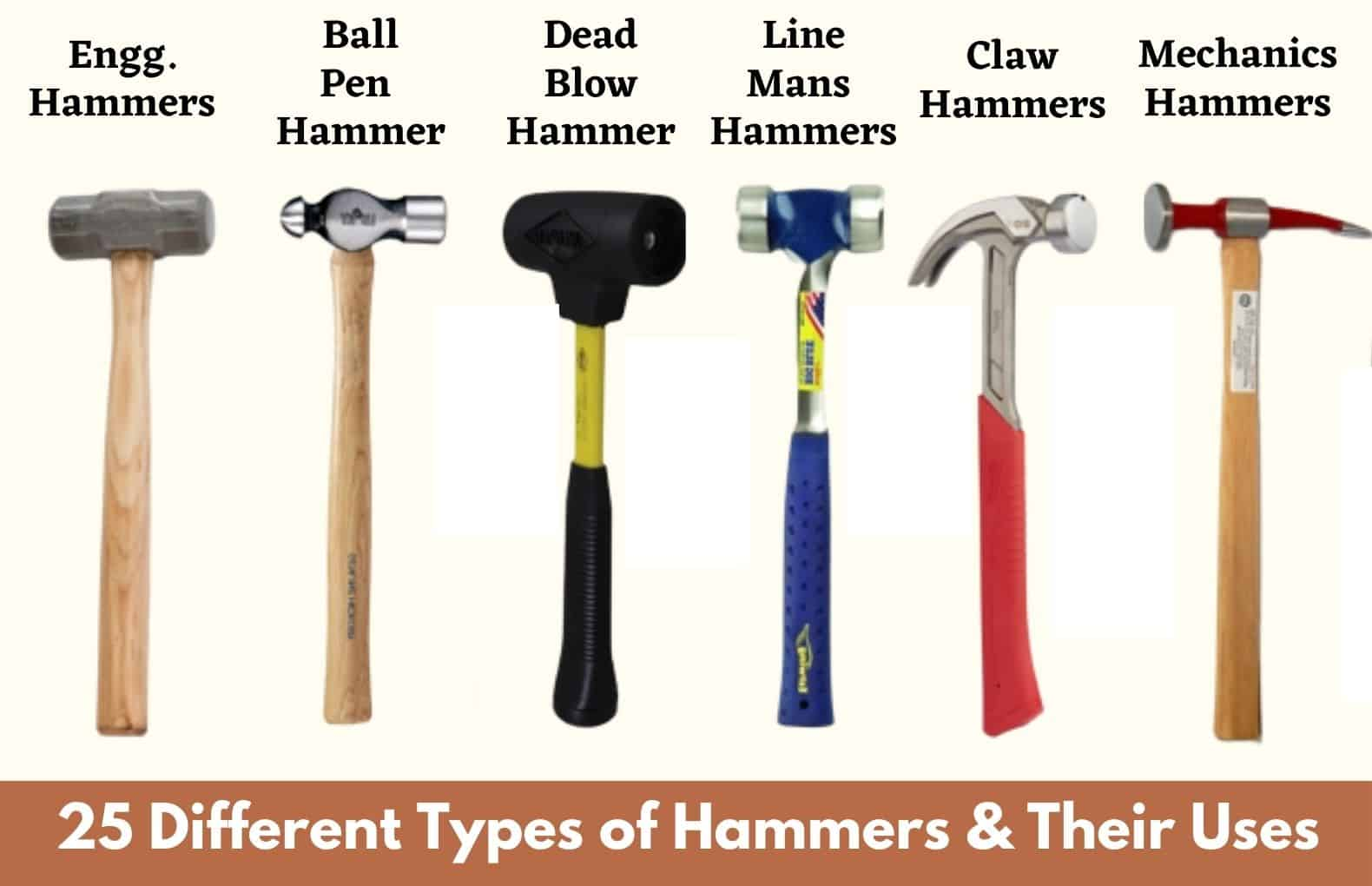#### 25 Types of Hammers | Different Types of Hammers and Their Uses | Top 25 Hammers Types#### 10 Different Types of Ladders and Their Uses | Best Types of Ladders | Material Used For Ladders#### 17 Types Of Contractors | What Is Contractor | 17 Different Types of Contractors | List of Contractors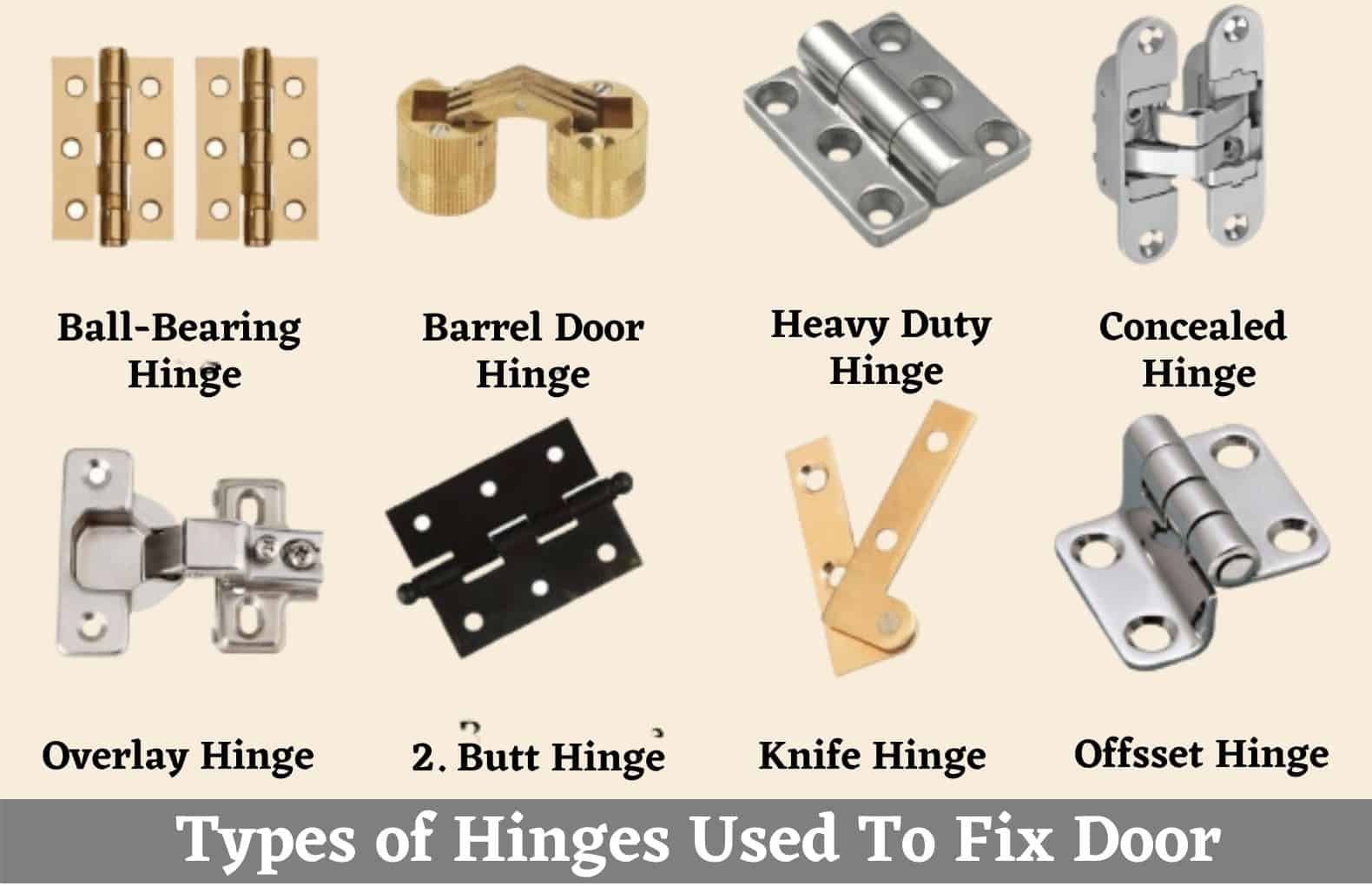#### 13 Types of Door Hinges | Types of Hinges Used For Door | Different Types Of Door Hinges | What Is A Butt Hinge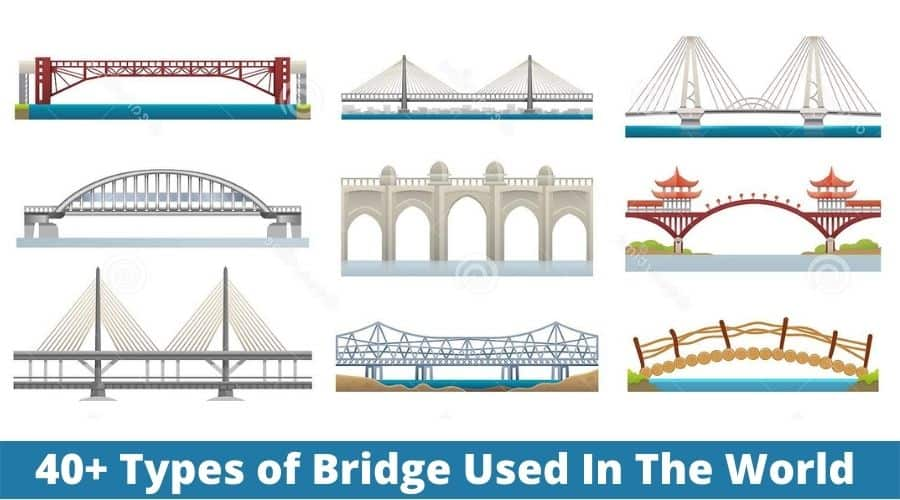#### 40 Types of Bridges | Classification of Bridges | 40 Different Types of Bridges Used In The World#### 10 Most Popular Types of Fencing | Types of Fence | Different Styles of Fencing | Fences for Yard﻿
x

### Related Posts

Foundation Materials List | House Foundation Materials | Materials Used In Foundation Construction
There are different types of Building Foundation Materials used to the foundation of the house. House foundation materials have prime importance...
Water Cement Ratio | Watercement Ratio | Water Cement Ratio Calculation Example | W/C Ratio Formula
What Is Water Cement Ratio? It Is the ratio of the mass of water to the mass of cement added to concrete. The Watercement Ratio directly affe...
What Is Concrete | Ingredients of Concrete | Concrete Test | Types of Concrete
What Is Concrete? Concrete is a composite material made by mixing different material like cement, sand, aggregate & water with or without...